Math Concepts

# Odd and Even Numbers

923 views

 1 Introduction 2 Identifying Odd & Even Numbers 3 Even & Odd (Parity) Properties 4 List of Odd & Even Numbers 5 Problem Solving & Solved Examples 6 Summary 7 FAQs

28th September 2020

## Introduction

A number that's divisible by 2 and generates a remainder of 0 when divided by 2 is known as an Even number. An odd number is a number that isn't divisible by 2. The remainder within the case of an odd number is 1.

An even number can be described as a number that may be divided into two equal groups. An odd number, on the other hand, can't be divided into two equal groups.

Even numbers end in 2, 4, 6, 8, and 0, no matter what preceding digits they have.

Odd Numbers ends in 1,3,5,7,9, no matter what preceding digits they have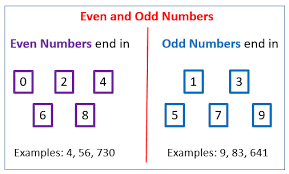## Identifying Odd & Even Number

### 1. By comprehending the amount at “ones” place

In this approach, we analyze the quantity at “ones” place in an integer to ascertain if the quantity is even or odd.

All the numbers that end with digits 1,3,5,7 and 9 are odd. For instance, numbers like 11, 23, 35, 47, etc. are odd numbers.

All the numbers that end with digit 0,2,4,6 and 8 are even numbers. For instance, numbers like 14, 26, 32, 40, and 88 are even numbers.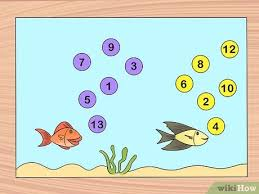### 2. By grouping

If we are able to divide digits into two groups with an equal number of elements in each, then the number is an Even Number. With the case of Odd numbers, we get a remainder of 1 while grouping.

### 3.As groups of “two” in each

For a number, if it forms multiple “two” groups with none remainder, it's an Even Number. Within the case of a remainder, the number is said to be an Odd number.

The given table explains the result once we apply different operations of two numbers.

(we know the number 5,917,624 is even because it ends with a 4!)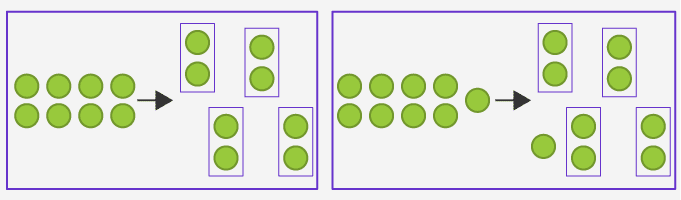### 4.As two equal groups

If we divide digits into two groups with an equal number of elements in each, then the number could also be an Even Number. Within the case of Odd  Numbers, we get a remainder of 1 while grouping.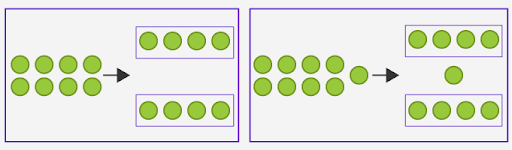## Even & Odd (Parity) Properties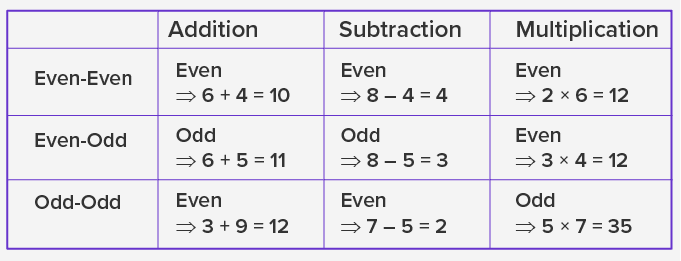The property by which we classify an integer in math as even or odd is additionally mentioned as parity.

An even number has parity 0 because the remainder upon division by 2 is 0, while an odd number has parity 1 because the remainder upon division by 2 is 1. For example, 0,2,4,10,-60,2,4,10,−6 are all even numbers because

they leave a remainder of 0 upon division by 2. The integers 1,3,5,11,-71,3,5,11,−7 are all odd numbers because they leave a remainder of 1 upon division by 2. Every digit can only be even or odd, and there's no number which

will have both even and odd. This includes 0, which is even.

The following are the parity properties of even and odd numbers: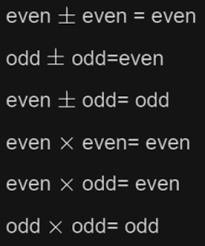These properties are often useful for testing whether equality is false or true by using arithmetic parity rules to determine whether all sides have the same parity.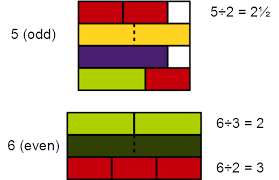## List of ODD  & Even Numbers

Even numbers are often divided evenly into groups of two. the Number 4 is usually divided into two groups of Numbers of 2(2+2)

Even numbers always end with numbers like 0, 2, 4, 6, or 8.

All these numbers like 2, 4, 6, 8, 10, 12, 14, 16, 18, 20, 22, 24, 26, 28, 30 are even numbers.

Odd numbers can't be divided into two groups.

Number 5 is often divided into two groups of two and one group of 1.

Odd numbers always end with numbers like  1, 3, 5, 7, or 9.

All these numbers like 1, 3, 5, 7, 9, 11, 13, 15, 17, 19, 21, 23, 25, 27, 29, 31 are odd numbers.

 1 Odd 2 Even 3 Odd 4 Even 5 Odd 6 Even 7 Odd 8 Even 9 Odd 10 Even 11 Odd 12 Even

## Practice Problems

 Example 1:

Are all whole numbers even?

### Solution:

 No, the list of whole numbers that are exactly divisible by two is named the even numbers.
 Example 2:

State Four consecutive even numbers between 11 to 19.

### Solution:

Let A = {11, 12, 13, 14, 15, 16, 17, 18, 19}

 Hence Number 12, 14, 16, 18 are Four consecutive even numbers.
 Example 3:

Choose the right answer. The sum of two even numbers

a) is usually a good number
b) is usually an odd number
c) is usually odd and sometimes even
d) could also be neither odd nor even

### Solution:

 a). Even number + Even number = Even number
 Example 4:

Find the sum of the smallest odd 3 digit number and largest 3 digit number and prove that it's divisible by 2.

### Solution:

The smallest 3 digits odd number is 101
The largest 3 digits odd number  is 999
Sum of these two numbers = 101 + 999 = 1100

 The number 1100 is divisible by 2 (as per divisibility rule of 2). This proves that the amount is even Number.
 Example 5:

Are all subsequent numbers odd?
25
15 + 13
32 – 37

### Solution:

25 isn't divisible by 2, so an odd number.
15 + 13 = 28, divisible by 2, not an odd number

 32 – 37 = -5, is an odd number

## Summary

How to Determine Odd and Even Numbers?

If the digit is evenly divisible by 2, it’s an Even number; otherwise, it’s an odd number. We can also say once we divide the number by 2 and there's some remainder left, which means that it isn't divisible again by 2, then it’s an odd number.

• Even + even = even
• Odd + odd = even
• Odd + even = odd

Multiplication

• The product of two even numbers is even.
• The product of two odd numbers is odd.
• The product of an Even number and an Odd number is Even.

## 1.How do you find odd numbers?

Odd numbers are defined as any number which can't be divided by two. In other words, digits within the sort of 2k+1, where k ∈ Z (i.e., integers) are called odd numbers. It should be noted that numbers or sets of integers on a number line can either be odd or even.

Some Important Points

1.An odd number is an integer which isn't a multiple of two
2.If these numbers are divided by 2; then there will be a remainder left
3.In the number line, 1 is the first positive odd number

## 2.How many odd numbers are there from 1-100?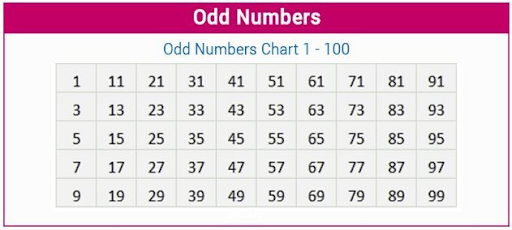## 3.How many Even numbers are there from 1-100?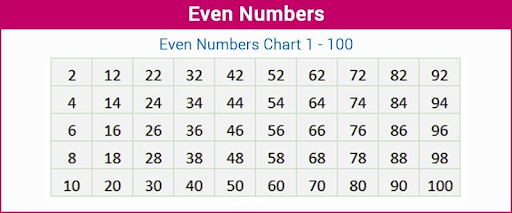Related Articles
GIVE YOUR CHILD THE CUEMATH EDGE
Access Personalised Math learning through interactive worksheets, gamified concepts and grade-wise courses